### MODEL QUESTION BANK | Diploma in Mechanical Engineering VI Semester | ESTIMATION & COSTING | mechanical engineering | DIPLOMA 5th and 6th Semester

MODEL QUESTION BANK

Diploma in Mechanical Engineering VI Semester

Course title: ESTIMATION & COSTING

 CO1: Understand the concept of estimation and costing and Analyse the concept of depreciation cost.

REMEMBERING

Chapter-I: Introduction to Estimation and costing

1.     Define estimation.

2.     List the functions of an estimator.

3.     Define costing.

4.     List the various costing methods

5.     What are the advantages of efficient costing?

6.     Define standard cost. Write the advantages of standard cost.

7.     Define the following terms

a.      Depreciation

b.     Estimation.

8.     Define obsolescence. List the various methods of calculating depreciation

9.     List the causes of depreciation

10.  Name six methods of calculating depreciation.

11.  List the aims of estimating

12.  List the essential qualities of an estimator.

UNDERSTANDING

10.  Explain the various qualities of estimator

11.  Explain the procedure for carrying out estimation.

12.  What are the sources of errors in estimating

13.  Explain the idleness in industries and list the various form of idleness.

14.  Explain the need of an estimation for industrial project

15.  Compare estimation with costing

16.  Explain the steps involved in estimating procedure.

17.  Explain the Straight line method of calculating depreciation

18.  Explain the Sinking fund method of calculating depreciation

19.  Explain the diminishing balance method for calculating the depreciation

20.  Explain the terms

a.      Repair and maintenance

b.     Obsolescence

21.  Explain the aims of estimation

22.  Explain on the various functions of an estimator

23.  Compare man hour rate with  machine hour rate

24.  Comparison depreciation with obsolescence

25.    Explain fixed instalment method of depreciation

APPLICATION / EVALUATING

9.     A machine was purchased for Rs 30000/-. The cost of erection charges was Rs 10000/- , the estimated life of the machine was 20 years. The scrap value at the end of useful life was Rs 8000/-. Determine the rate of depreciation per year by straight line method. After 10 years the machine underwent major repairs and the repair cost was Rs 6000/- .What will be the new rate of depreciation per year?

.

10.  The installation cost was Rs 11950/-. The apparatus has a life expectancy of 20 years with a scrap value of Rs 6000/-. What should be the rate of depreciation and depreciation fund on 1.1.1990 by reducing balance method?

11.  A lathe is purchased for Rs 80000/-. The assumed life is 10 years and scrap value Rs 8000/-. If the depreciation is charged by diminishing balance method. Determine the rate by which the value of lathe is reducing every year and Estimate the depreciation fund after 2 years.

12.  A machine is purchased for Rs 60000/-. The estimated life of machine is 15 years and the scrap value is Rs 15000/-. Determine the depreciations per year by using interest law (Sinking fund) method, if the rate of interest is charged at 5%. Estimate the depreciation at the end of 5 years.

13.  An industrial plant with initial value of Rs. 400000/- has a salvage value of Rs.50000/- at the end of 25 years, but sold for 260000/- at end of 10years. What is the profit or loss to the owner, if sinking fund method of depreciation is adopted? Take interest rate @ 8%.

14.  A machine was purchased for Rs.40000/- the useful life of machine is 15 years. The scrap value of the machine is Rs.1000/- . after 5 years, the machine was sold for Rs.24000/-. If depreciation was set aside by sinking fund method, the rate of interest @ 8%, determine the extra capital needed to purchase the new machine for Rs.50000/-.

15.  The cost of the machine is Rs. 16000 and its scrap value is Rs.4000/-. Determine the

depreciation by “sum of the years digits method “charges for the year, if the estimated life of the machine in 4 years.

16.  Determine the depreciation at the end of each year by the sum of years digits method (SYD), if the life of the plant is 5 years. The assets of the company are Rs.360000/- and scrap value is Rs.60000.REMEMBERING

1.      List out the various elements of cost

2.      Name the various components of cost.

3.     List the various indirect expenses.

UNDERSTANDING

4.     Compare direct expenses with indirect expenses.

5.     Comparison fixed with variable overheads.

7.     Explain the following a) personal allowance b) fatigue allowance

8.     Explain the control on prime cost, control on overheads and control over indirect materials and tools.

9.     Explain the unit rate method designed for allocation of on cost

10.  Explain each element of cost with suitable examples

11.  Explain each components of cost with suitable examples

12.  Illustrate various elements of cost in determining the price of a product with the help of a block diagram.

13.    Explain a) Prime cost b) Direct material Cost c) Direct labour cost

14.  Explain the method involved in calculation of direct material cost.

APPLICATION/EVALUATION

1.     The direct material used is Rs 1000/- and the direct wages are Rs 443/- for the manufacture of certain items. Determine the factory cost, when the oncost is to be 60% of prime cost.

2.     A certain articles are manufactured in bathes of 100. The direct material cost is Rs. 250/- , direct labour cost Is Rs.400/- and factory overheads is Rs. 290/-. If the selling on cost is 40% of prime cost. What should be the selling price of each product to obtain a profit of 20% on the selling price?

3.     A machine is manufactured by a firm in batches of 100. The direct material cost is Rs 1600/- and direct labour cost is Rs 2000/-. Factory on cost is 30% of the prime cost. Overhead charges are 20% of factory cost. If the management wants to make a profit of 10% on the selling price. Determine the selling price of each machine.

4.     A concern produces bolts & nuts of aluminium and estimated cost is given on the basis of a lot of 1000 bolts with nuts, length of bolt with head 10cm and diameter 2cm.

i)  Material cost Rs 700

ii)  Labour cost (direct)

a)  Cutting and setting up =Rs 150

b)  Milling, threading, drilling = Rs 160/-

iii)  Cost of tools            =Rs 100/-

iv)  Factory overheads    =150% of total labour cost

v)  Office on cost           = 30% of factory cost

vi)  Selling price             = Rs 2/ piece

Determine whether management is making profit or loss per piece.

5.     Estimate the total cost of two products A & B of on cost is 60% of prime cost. The product has Rs 200/- as direct material and Rs 400/- as direct labour cost, While the product B has Rs 300/- as direct labour cost and Rs 300/- as direct material cost.

6.     A lot of 5000 pieces of an item was fabricated and the following cost were incurred

·       Material cost     Rs 1000/-

·       Labour cost       Rs 1100/-

·       Cost of tool/etc Rs 125/-

·       Factory oncost 150% labour cost

·       Office oncost    25% of factory cost

·       Selling expenses 20% of oncost production

What should be the profit or loss if the price of each item is fixed at Rs 1.50

7.     A catalogue price of a machine is Rs 6000/- and the discount allowed to the distributors is 20% of the catalogue price. At some stage, it was found that the selling expenses were half the factory cost and material cost, labour cost and the factory overheads were in the ratio of 1:4:2 if the material cost was Rs 400/-. What profit was made by the factory on each machine.

8.     A market price of a machine is Rs 50000/- and the discount allowed to the distributor is 20% of the material price. Selling expense cost is ¼ of the factory cost. If the material cost,  Labour cost and factory overhead charges are in the ratio of 2:4:1, What profit is going to be made by the factory on each machine, if the material cost is Rs 8000/-.

9.     An article can be made either by hand or in large quantities by mass production. In the farmer case, time taken is 3hrs and overheads is 25% of labour cost. While in the later case time taken for 10 pieces is 8Hrs, but overheads are 150% of the labour cost, material cost is Rs 15/ piece and labour charges are Rs 8/Hr. Compare the total cost in both the cases. Which is cheaper?

10.  A factory owner employed 50 workers during the month of march 2000. Whose details of expenditure are as given below: a) Material cost is Rs 100000/- b) Rate of wages for each worker is Rs 6/Hr c) Duration of work is 8hrs/day d) No. of holidays in the month is 6 days and e) Total overhead expenses is Rs 50000/-. If workers were paid overtime of 200Hrs at the rate of Rs10/Hr. Determine i) Total cost ii) Man hour rate overheads

11.  Estimate the weight of cast iron used in manufacturing step pulley as show in figure. Assume density of CI as 7.2 gm/cc.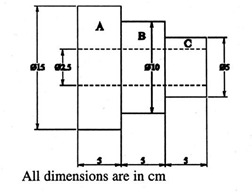12.  Determine the weight and cost of material of MS spindle as shown in figure. The density of MS is 7.8 gm/cc and rate is Rs.15 per Kg.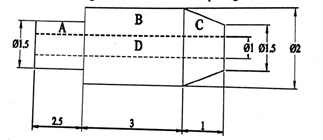13.  Determine the number of rivets of dimensions as shown in the figure. Which can be manufactured from 4 Kg of MS. Assume that there is no wastage of material density of MS is 8 gm/cc. All dimensions are in cm.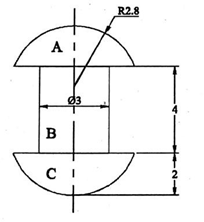14.  Figure shows a lathe centre. Evaluate the weight and cost of the material which weighs 7.85 gm/cm3. The cost of the material is Rs. 20 per kg.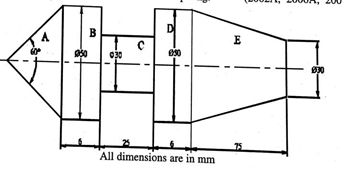15.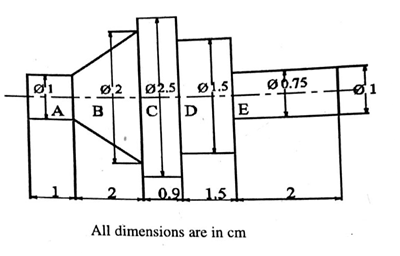Estimate the volume of the material required for manufacturing 100 pieces of shaft shown in figure. The shaft is made-up of MS weights 8 gm/CC and cost is Rs.60 per kg. Determine the material cost for such shafts.

16.  Determine the weight and cost 200 articles of MS as shown in figure. Assuming suitable density and the material cost as Rs.12 per Kg.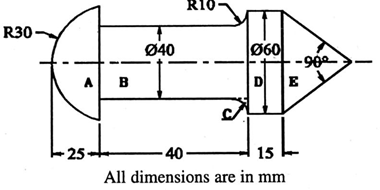17.  Determine the material cost of ten gun metal bushes of 15 cm length including flanges at both the internal dia. Of the bush is 4 cm, external dia is 8 cm and outer dia of the end flanges is 12 cm. assume density of metal as 8.5 gm/cc and cost of the material is Rs.200/kg.

18.  Determine the weight of the gear blank of CI as shown in figure. Taking its density as 7.4 gm/cc.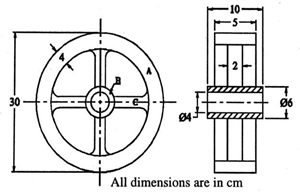19.  Estimate the material cost of an engine flywheel from the following data:

 Inside dia of the flywheel rim =70 cm Outside dia of rim =100 cm Width of rim =25 cm Inside dia of hub =12 cm Outside dia of hub =24 cm Length of hub =30 cm Section of arms (elliptical) =7 cm X 4 cm Length of arm =23 cm No. of arms =4 Density of material =8 gm/cc Material cost = Rs.25/kg Neglect tapering of arms .

CO3: Ability to estimate the various machining operations

REMEMBERING

1.                   List the various operations performed in machine shop.

2.                  Define cutting speed, feed, depth of cut for lathe operations

3.                  Define cutting speed, feed, depth of cut in lathe

UNDERSTANDING

1.                     Explain the various operation time to be considered for estimation of Machining time.

2.                     Explain the Procedure of estimating cost of machined part for Lathe operations

3.                     Explain the Procedure of estimating cost of machined part for Milling operations

4.                     Explain the Procedure of estimating cost of machined part for Cylindrical Grinding operations.

APPLICATION

1.     Estimate the time required to turn 3.5 cm dia bar to dimension shown in figure. Cutting speed is 15.4 m/min and feed is 1 mm/rev. all cuts are 3.5 mm deep.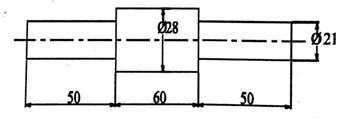2.     (a) How much time will be required to reduce a 42mm bar to dimension shown in figure. With cutting speed 16.5 m/min and feed is 1 mm/rev. assume all cuts are 2.5 mm deep. (b) How long will it take to machine a 45o X 3.125 mm chamfer?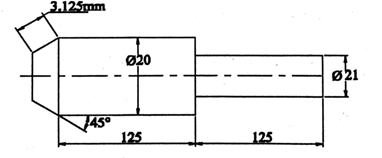3.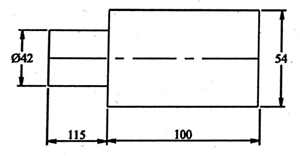Estimate the time required to run a 60mm dia rod to the dimension shown in figure. Taking cutting speed as 20m/min, feed as 1.2 mm. all cuts are 3mm deep. All dimensions are in mm.

4.     A tapered component is to be manufactured in a lathe by compound rest method out of a given bar of 60mm dia and 100 mm length. Assume the following data:

Details of tapered components Major dia =60mm

Minor dia =20mm Length of taper =100 mm

Cutting speed 75 m/min. hand feed by compound rest =0.05 mm/rev depth of cut should not exceed 4 mm. Determine the time required for machining. All dimensions are in mm.

5.     A component shown in figure is to be knurled on the surface. Estimate the time required for knurling it, if the cutting speed is 20m/min and feed is 0.03 cm/rev.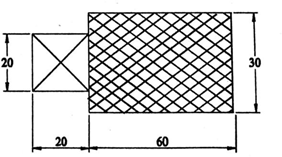6.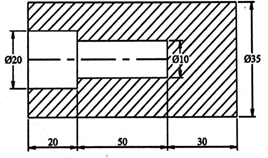Determine the time required for drilling component as shown in the figure. Assume cutting speed as 22 m/min and feed as 0.02 cm/rev. all dimensions are in mm.

7.     Estimate out the time for threading on a 3 m dia aluminium bar of length of 10cm by single point tool, if 3threads/cm are to be cut and speed bar is 80rpm. Assume suitable approach over run and no. of cuts.

8.     Determine the time required for cutting two threads/cm on aluminium bar stock of 3.5 cm diameter and for a length of 10cm. assume cutting speed as 11m/min. neglecting approach and overtake for the tool.

9.     Estimate the time required for tapping a 2cm dia. Hole with a 3 mm pitch tap in a MS plate upto a depth of 3cm. assume cutting speed as 10m/min and return speed of tap as 2 times the cutting speed.

10.  Estimate out the time required for tapping a 2.1 cm dia hole with 2.5 mm pitch tap in a mild steel plate upto a depth of 3.5cm. assume cutting speed as 11m/min and also assume that return speed of tap is twice the cutting speed. No. of cuts required is 6.

11.  What is the feed per tooth of a 32 teeth milling cutter of 40 cm dia , having a speed of 75 rpm, table feed 30per min. also Estimate the time required to face mill a CI casting 1.5 m long and 24 cm wide.(take allowances into consideration).

12.  Estimate the time required to face a job 20 cm lomg and 10 cm wide with the help of milling cutter of 10cm dia, having 8 teeth and revolving at 80 rpm. The feed per tooth should not exceed 0.125 mm. assume that the width of the cutter is sufficient to mill the whole of the job at a time.

13.  A 20 X 7.5 cm CI surface is to be faced on milling machine with a cutter having a diameter of 125 cm and 20 teeth. If the cutting speed and feed are 50m/min and 4.5 cm/min respectively, determine the milling time, rpm of the cutter and feed per tooth.

14.  A slot is tobe made on a milling machine with the help of a cutter, revolving at 100 rpm. Finf the time required to prepare the slot in two cuts, if it is 15 cm deep and 20 cm long with a cutter of 10 cm dia. Assume feed as 0.5 mm/rev.

15.  A slot of length 25 cm and of depth 3.375 cm is to be milled by 12.5 cm diameter cutter. What is the total table travel to complete the cut? Also Estimate the time required for milling the slot when the cutter is turning at 191 rpm with an average feed of 0.0125 cm and having 16 teeth.

16.  Estimate the time required to cut 60 teeth on a gear blank of 50 mm thick, with a feed of 30 mm/min. take added table travel as 10 mm and setup time as 10 minutes.

17.  Estimate the time taken to prepare a square prism on a milling machine from a round block 90 mm length and 40 mm dia. Feed 25mm/min. setup and indexing time is 15 minutes.

18.  Determine the time taken for shaping a cast iron block 50 cm long and 30cm wide in a single cut. Feed is taken to be 0.8 mm/ stroke and cutting speed 1 m/min.

19.  Estimate the time required for planning a piece of mild steel75 cm long and 30 cm wide on a planning machine. The cutting speed on the tool is 10 m/min and feed 0.0782 cm/ stroke.

20.  A machine bed is to be planed in two cuts on a planning machine. It takes 10 seconds in forward stroke and 7 seconds in return stroke. Estimate out the time required to plane the bed of width 40cm. assume the feed as 1mm/stroke.

21.  Estimate the time required for doing rough grinding of a 16cm long steel shaft to reduce its dia from 4.2 to 4 cm in a grinding wheel of 12 cm face width. Assume cutting speed as 16.5m/min and depth of cut as 0.25mm.

22.  The top of a cast iron table of size 40 X 9 cm is to be ground by a wheel having 2 cm face width. If the feed is 1/4th of the width of the wheel and the table moves 9m in one minute. Estimate out the time required for grinding in two cuts.

23.  Estimate out the machining time to complete the job as shown in figure. From the basic raw material of 50 mm dia and 100 mm length. Cutting speed for turning 30 m/min, feed=1mm/rev, depth of cut=2.5mm. cutting speed for thread cutting=9 m/min. cutting speed for drilling=30m/min and feed for drilling=0.2 mm/rev.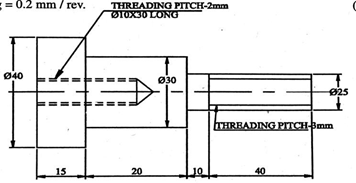24.  Estimate the time taken to prepare a job according to the dimensions shown in figure. From a bar 3.5 cm dia and 6 cm long. Assume following data.

Cutting speed for turning and boring                                                            = 20m/min Cutting speed for drilling                                                  =30m/min

Feed for turning and boring                    = 0.2mm/rev

Feed for drilling                                    = 23 mm/rev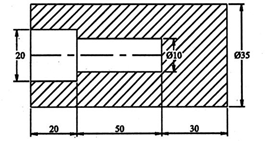Depth of cut not to exceed                     = 3mm.

25.  It is desired to manufacture 100 bolts/day from MS bar of length 10 cm and diameter 30 mm. bolt has the following dimensions: dia of shank=1.2cm, dia of head =20 mm, thickness of head=10mm, lengt of bolt (face to face) = 10 cm. length of threaded portion = 3cm. assume

i)  feed for turning =0.2cm/rev

ii)  cutting speed for turning =20m/min

iii)  cutting speed for threading    = 10m/min

iv)  depth of cut                          = max. of 3mm

v)  pitch of threads                     = 2.5 mm

vi)  no. of cuts required for threading =6

Determine the machining time for 100 bolts if the operator is paid at the rate of Rs.

4.25 per hour. Estimate the labour charges considering other allowance for fatigue etc as 100%.

CO4: Ability to estimate the various welding & sheet metal components

UNDERSTANDING

1.          Explain the any five sheet metal operations.

2.          Explain the types of sheet metal joints.

3.          Explain types of welding.

4.          Explain the types of welding costs.

5.          Explain the factors affecting the welding cost.

APPLICATION

Estimation in welding shop

1.     Estimate the time required for making an open tank of size 50x50x50 cm by gas welding. Size of sheet used is 50x50x0.3 cm welding is to be done on both sides. Assume fatigue allowance to be 10% and welding speed outside as 6m/hr and inside 5m/hr.

2.     Estimate the material cost for linear welding of two flat pieces of MS 45cm x 6 cm x 1 cm size at an angle of 90o by gas welding on both sides. Neglect edge preparation cost and assume i) cost of oxygen                                    =Rs 10/m3

ii)  cost of acetylene       =Rs 60/m3

iii)  density of filler metal=7 gms/cc

iv)  cost of filler metal = Rs 12/kg from the table for 10mm thick plates

i)  O2 consumption =0.7 m3/hr

ii)  C2H2 consumption= 5mm

iii)  filler rod dia          = 5mm

iv)  length of filler rod required=4.5 m/m of welding v)welding time                              = 30 min/m of welding

3.     Estimate the welding material cost for making a rectangular frame for a gate of 2m x 1m from angle iron size 30mm x 30mm x 5mm. assume the following data:

i)  oxygen consumption=0.4 cu.m/hr which is available at Rs. 50/cu.m

ii)  acetylene consumption = 0.4 cu.m/hr which is available at Rs.50/cu.m

iii)  welding speed          = 4 m/hr

iv)  length of filler rod of dia 2.5mm= 3.4 m/m of welding

v)  filler rod material cost           = Rs.13/kg

vi)  welding is to be done on both sides

4.     A cylinder boiler drum 3m x 1m dia is to be made from 1.5 thick MS plates. Both the ends are closed by welding circular plates to the drum. The cylindrical portion is welded along the longitudinal seam both inside and outside. Circular plates are welded only on outside. Determine the cost of electric arc welding using the following data:

i)  rate of welding 2m/hr on inside & 2.5 m/hr outside

ii)  length of electrode required 2m/m of welding

iii)  cost of electrode Rs. 6/- per meter

iv)  power consumption 5 kwh per metre of weld

v)  power charges Rs 2/- per unit

vi)  labour charges Rs 10/hr

vii)  overhead charges 200% of prime cost

ix)  fatigue 7 setting up time -5% of welding time

5.     Two 1m long MS plates of 10mm thickness are to be welded by a lap joint with the help of a 6 mm electrode. Determine the cost of welding

a.      Current used                :250 amp

b.     Voltage                                   : 30 V

c.      Welding speed                         : 10m/hr

d.     Electrode used                         : 0.4 kg/m of welding

e.      Labour charges Rs 1/ hr

f.      Power charges Rs 0.20/kwh

Cost of electrode Rs 5/kg

6.     A container open on one side (top side) of size 0.5x0.5x1 m height is to be made from 5mm thick plate. Take the density of plate metal as 8 gms/cc. the joints one to be welded. Estimate the cost of the container from the following data

Cost of plate =Rs. 12 per kg Sheet metal scrap = 5% of the material

Cost of labour                                    =10% of material cost Cost of welding material=Rs.30 per metre of weld

7.     An open water tank 1mx1mx2.5m height is to be favricated from MS plates of 2.5cm thick. Estimate the cost of the tank from the following data.

Density of MS               =8gm/sec

Cost of MS plate           =Rs. 6/kg

Cost of fabrication                                    =25% of material cost Cost of welding                                    =Rs. 0.25 per cm length Factory overhead charges= 30% of prime cost

Sales overheads                                    =20% of manufacturing cost Profit                            =25% of total cost.

8.     A mild steel vessel 1m in dia and 2m in length is to be fabricated by using MS sheets of 5mm thick by welding on both sides. Sheets are available in sizes 1mX3m. estimate how many number of sheets required and the total quantity of the material required. Also Estimate, welding length, length of electrode, time required for welding for welding and power consumption. Use following data: density of MS=7.8 g/cc, rate of welding=1.5m/hour. Length of electrode=1.5m/m of weld, power consumption=4kwh per m of weld. Illustrate clearly with appropriate sheet layout to support your estimation.

9.     Estimate the cost of metal sheet for preparing a funnel as show in figure. Assume the wastage of metal as 10% and cost of sheet as Rs.50/m3.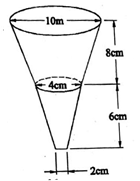10.  Estimate the cost of metal sheet for preparing a funnel as shown in figure. Assume the stage of metal as 10% and cost of the sheet as Rs. 20/m2 . all dimensions are in mm.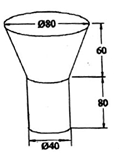11.  A cylinder drums of size 2.75 m height and 1.5m mean dia are to be fabricated from sheet of 5mm thickness by grooved seam joint and both the ends should be closed by sheet with single seam joint. Determine the material cost, if sheet is available at Rs. 2000/ m2.

CO5: Ability to estimate the various forging & foundry shop components.

UNDERSTANDING

1.     Explain the Cost terminology associated with forging shop.

2.     Explain the procedure of calculating material cost of a product for forging shop

3.     Explain the types of forging.

4.     Explain the Procedure for estimating forging cost.

5.     Explain the forging losses to be considered while estimating.

6.     Explain the pattern allowances.

7.     Explain the procedure for calculating material cost of a product for foundry shop.

8.     Explain the procedure for estimating cost of pattern making.

APPLICATION

1.     Prepare a hexagonal bolt 15mm diameter and 25cm length from a bar stock of 1.8 cm diameter. Determine the length of the bar stock required and also give the sequence of operations for forging the above bolt.

2.     Estimate the size of stock and weight of material required to forge 100 MS bolts as shown in figure. The bar stock diameter is 3 cm.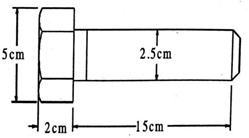3.     A hexagonal bolt 25mm dia and 200 mm length is to be forged from a bar stock of 30 mm dia. Determine the length of bar stock required if the total material loss is 15% of the net weight of the bolt. Hexagonal bolt head has 20 mm thickness.

4.     Determine the gross weight of the MS bolt if it is produced in a lot of 5000. If steel weighs

7.9 gm/cm3 and the method is upsetting. Also Determine the length of the bar required. Take spoilage loss as 10% of net weight, loss of scaling to be allowed as 5% of each component. The bolt is square headed bolt with head 40 square and height 15 mm, shank dia is 20mm, length 55mm. all dimensions are in mm.

5.     Determine the length of 10 mm dia rod required to forge a ring of 50 mm inner dia. A scale loss of 5%.

6.     A square bar of 3cm side and 25 cm length is to be converted by hand forging into a bar of hexagonal section having each side equal to 1.5cm. Determine the length of hexagonal bar produced; consider scale loss to be 7% of the total volume.

7.     Estimate the length and weight of 1 cm dia stock required to hand forge 200 rivets of dimensions given in figure. Assume density of material as 8 gm/cc.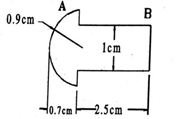8.     It is required to make a key of dimensions given figure. From a rod of 20mm dia. Determine the length of rod required on forging.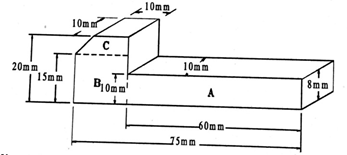9.     300 pieces of gib headed key shown in figure are to be drop forged from a 4cm diameter bar.

Determine the cost of material if

i)  material cost is Rs. 50/- per metre

ii)  scale loss 8%

iii)  shear loss as 3 mm length/piece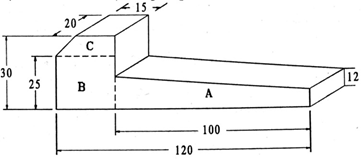10.  A finished drawing of a CI flanged pipe is given in figure. Your are required to prepare a wooden pattern in the pattern making shop. Estimate the quantity of wood required for manufacturing the pattern. Also estimate the cost of pattern if:

a)  wood is available at the rate of rs.2800 lm3

b)   pattern maker is available at the rate of Rs 80/ day and takes 6 hrs in preparing the pattern

c)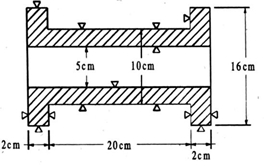overhead charges are 20% of material cost.

11.  Estimate the cost of 2000 CI pulleys as shown in figure. Its surfaces are to be machines after casting. The pattern is supplied by the customer itself. Following data can be used:

Cost of metal = Rs.5/kg Moulds prepared by each worker/day=20

Melting charges            = 20% of metal cost

Machining allowance on each side may be taken as 2mm Wages of each moulder =Rs. 16/day

Density of CI = 7.2 gm/cc Overhead charges = 25% of metal cost.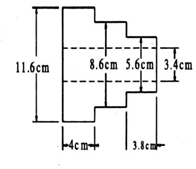12.  A manufacturer is willing to undertake the casting of 100 CI wheels shown in figure. Estimate the selling price of each wheel assuming the following:

i)  cost of pattern            =Rs.40

ii)  process scrap            = 10% of net weight

iii)  scrap return value     = Rs.2.25/kg

vi)  selling overheads     = 25% of production cost

vii)  profit                      = 15% of total cost

 unit operation time Rate/hour Shop overheads moulding 10 min Rs. 3 Rs. 10/hr pouring 6 min Rs.2.50 Rs.8/hr shot blasting 4min Rs. 4.50 Rs.3.50/hr fettling 5 min Rs. 2.50 Rs. 3.00/hr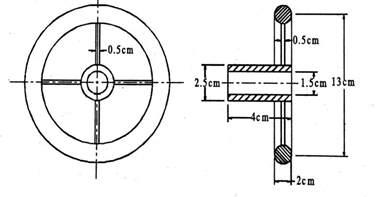UNDERSTANDING

1.       Explain the process costing.

2.       Explain the characteristics of process costing.

4.       Explain the principles of process costing

5.       Differentiate between job costing and process costing

6.       Explain the procedure of process costing

7.       Define a) nominal wages b) real wages c) fair wages d) minimum wages

8.       Explain the concept of incentives

9.       Explain different types of budget

10.   Explain briefly: book value-Net Present Value-Work in progress- Gross Domestic Product (GDP)-balance sheet

11.   Explain manual tendering

12.   Explain E-tendering